Name:    Quiz B.4-B.7

Multiple Choice
Identify the choice that best completes the statement or answers the question.

Use a number line to find the sum or difference.

1.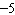+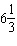a.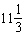c.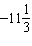b.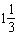d.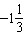2.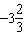+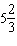a.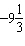c.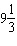b.d.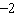3.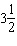a.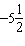c.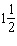b.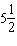d.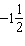4.

–1 – 3.4
 a. 4.4 c. –2.4 b. 2.4 d. –4.4

5.

9 – 3.2
 a. –5.8 c. 12.2 b. 5.8 d. –12.2

6.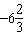+a.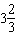c.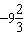b.d.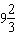7.

How many quadrants are in a coordinate plane?
 a. 3 c. 2 b. 1 d. 4

Write the ordered pair that corresponds to the point.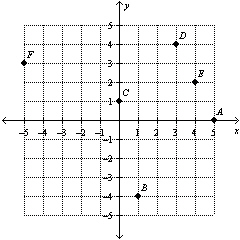8.

Point A
 a. (5, 0) c. (–5, 0) b. (0, 5) d. (0, –5)

9.

Point B
 a. (1, –4) c. (–4, 1) b. (4, –1) d. (–1, 4)

10.

Point C
 a. (0, –1) c. (1, 0) b. (–1, 0) d. (0, 1)

Numeric Response

Use a number line to find the sum or difference.

1.

0 + 6.3

1.

Two vertices of triangle ABC are A(–3, –3) and B(7, –3). List four possible coordinates of the third vertex so that the triangle has an area of 25 square units.

Write an equation for the function shown in the table.

2.

 Input, x –3 –2 –1 0 1 Output, y –9 –8 –7 –6 –5